# PHP Control Flow: Loop Types and Conditional Statements ExplainedEach programming language constitutes a basic set of rules that define the flow of control in a program based on certain conditions. They specify the sequence and order of execution of instructions in your code.

These control structures lie at the core of programming paradigms and are usually the first things you’ll come across when you learn a new programming language. They can be broadly classified into two categories - Repetitive Flow (Loop-based) and Conditional Flow (If-else based). Loops allow you to perform repetitive tasks in your code whereas conditional statements can be used to run different parts of your code based on if a condition is met or not.

In this post, we’ll look at the different ways in which we can leverage these various control structures in PHP. We’ll look at different types of loops and conditional statements, and explore how we can use them in defining logic for our code.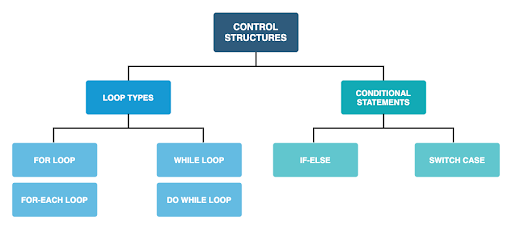For Loops

Nesting For loops

Breaking out of a loop

Continue to the next loop iteration

For-Each loop

While Loop

Do-While

Conditional Statements in PHP

Switch-case

Return statement

Putting it all together

## For-Loops

For loops are the most common loops in programming. For people starting out with programming, For loops can seem a little abstruse at first, especially in languages like C, C++, PHP, etc. But once you get the hang of them, they’re quite easy to implement.

### How to Do For Loops in PHP

This is what the template of a For loop in PHP looks like -

``````for (init; condition; update) {
#statement
}
``````

The way that this work is -

• init refers to an initialization statement that is executed only once, at the beginning of the loop. This is most commonly used to initialize an iterator that keeps track of your loops.
• Before each loop iteration, the condition is evaluated. The loop proceeds to the body’s execution if the condition is true and terminates if not. This condition usually involves the initialized expression to evaluate whether to continue looping or to exit the loop.
• the update refers to the expression that is executed after every loop iteration. It is mostly used to update the initialized iterator.

Let us understand this using an example.

### PHP For Loop examples

Let’s see how we can use a for-loop to print whole numbers from 0 to 5 in PHP.

``````<?php

for (\$i=0; \$i<=5; \$i++) {
echo \$i." ";
}

?>
``````

In the above example, we see that \$i is used as an iterator. We also note the following things-

• init expression: `\$i=0`

This implies that at the beginning of the execution of the loop `\$i `is initialized to 0.  `\$i `will be used as an iterator for our loop here.

• condition expression: `\$i<=5`

This implies that the loop will continue as long as `\$i<=5 `and will terminate as soon as the condition is not true.

• update expression: `\$i++`

This implies that at the end of each iteration, `\$i++ `is executed i.e. the iterator` \$i `increments by 1.

OUTPUT:Now let’s use a for-loop to traverse and print the elements of a PHP array. To do so, we will be iterating over the indices of the array elements and print the corresponding array values.

``````<?php
\$myarray = array('Hello', 'world', '!');
for (\$i=0; \$i<=count(\$myarray); \$i++) {
echo \$myarray[\$i]." ";
}
?>``````

OUTPUT: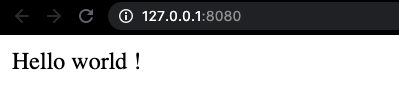Note: The count() function can be used to get the length of an array.

### Nesting For Loops

Sometimes you might want to run a loop inside an existing loop, for example, to print the elements of a multidimensional array. This can be achieved by placing loops into one another, allowing you to iterate along multiple dimensions. This is also known as nesting of loops.

``````<?php

\$myarray = array(
array('Hello', 'world', '1'),
array('Hello', 'world', '2'),
array('Hello', 'world', '3')
);

for (\$i=0; \$i<count(\$myarray); \$i++) {
for (\$j=0; \$j<count(\$myarray[\$i]); \$j++) {
echo \$myarray[\$i][\$j]." ";
}
echo "<br>";
}

?>``````

In the above example, you can see how nesting loops allow us to traverse across two dimensions - 1) across multiple arrays (using \$i) and 2) across multiple elements in each array (using \$j).

OUTPUT: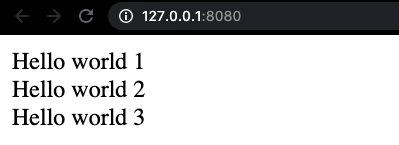You can nest your loops any number of times as per your requirement.

### Breaking out of a loop

Sometimes you might want to get out of a loop mid-way after you’ve got the desired result. You can choose to terminate the loop in the middle of it’s execution, whenever you like by using the break statement.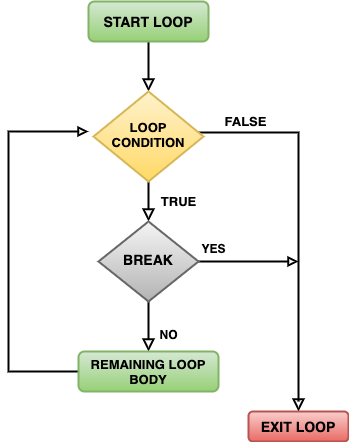The break call terminates the loop execution and allows control to get out of the enclosing structure. It can also be used with the other loop types that we will see later in the post.

Let’s understand how break works through an example.

We’ll create an array of strings, and search for an element in the array. As soon as we find the required element, we need not continue traversing the array and can therefore break out of the loop. Let’s see how this can be done.

``````<?php

\$myarray = array("Python", "HTML", "PHP", "Javascript",  "Ruby");

for (\$i=0; \$i<count(\$myarray); \$i++) {

if (\$myarray[\$i] == "PHP") {
echo "Found PHP! Breaking out of the loop.";
break;
}
else {
echo "Didn't find PHP. Continue looping ..";
}

echo "<br>";
}

?>``````

OUTPUT: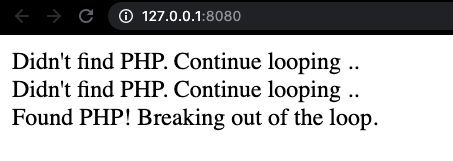In case of nested loops, if you want to break out of multiple nested enclosures you can use the optional numeric argument to specify the number of enclosures you want to break out of. For example, you can use break 2; to get out of a double-nested loop.

The default value of the argument is 1, so if not specified, the break command gets you out of the immediate enclosure.

### Continue to the next loop iteration

In the previous section, we saw how we can use break to get out of the enclosing loop immediately. In some cases, instead of breaking out of the whole loop, you might want to skip the remaining part of the current loop iteration and jump to the next iteration (if any iterations are left).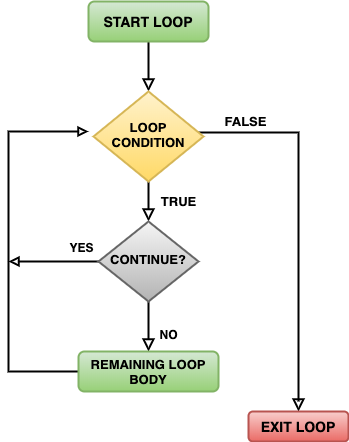This can be achieved using the continue call. Let us understand this using an example.

We’ll create a program that prints all characters of a string except for ‘l’.

One way we can do this is by using continue to skip the printing section of the code if the character ‘l’ is encountered, and move to the next iteration.

``````<?php

\$mystring = "Hello world!";

for (\$i=0; \$i<strlen(\$mystring); \$i++) {

if (\$mystring[\$i] == 'l') {
continue;
}

echo \$mystring[\$i];
}

?>``````

Note: The
strlen() function can be used to get the length of a string.

OUTPUT: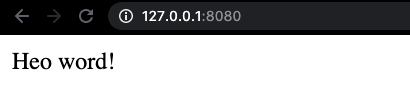## For-each Loops

Traversing arrays using for loops can seem a little cumbersome.
Why specify three expressions to keep track of the iterator when all you want to do is traverse the elements of an array?

### How to do For-Each loops?

To make it easy to traverse arrays, one can use PHP’s For-each loops. As we’ll see, For-each loops are syntactically way more easier to deal with, and therefore a better option for traversing arrays.

This is what the template of a For-each loop looks like.

``````foreach (\$myarray as \$element) {
# statement;
}``````

On each iteration, the value of the current element of \$
myarray is assigned to \$element.

### PHP For-Each loop example

Let’s print the elements of an array using the For-each loop.

``````<?php

\$myarray = array('Hello', 'world', '!');
foreach (\$myarray as \$elem) {
echo \$elem." ";
}

?>``````

OUTPUT: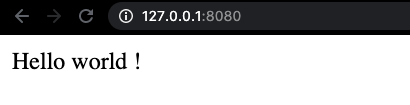In some cases, you might also want to use the indices of an array or keys of an associative array. This can be achieved using the following syntax -

``````foreach (\$myarray as \$key => \$value) {
# statement
}``````

On each iteration,
\$key is assigned the index (or key) of the current element and \$value is assigned the value current element of the array.

Let’s traverse an associative array using a For-each loop.

``````<?php

\$myarray = array("one"=>1, "two"=>2, "three"=>3);
foreach (\$myarray as \$key=>\$value) {
echo \$key." : ".\$value."<br>";
}

?>``````

OUTPUT: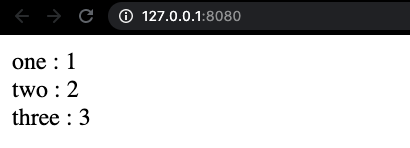## While loops

While loops are the simplest loops to define. When programming a while loop, all you need to do is define the loop condition. This condition is evaluated each time before running the loop. As long as the condition is true, the loop continues to run.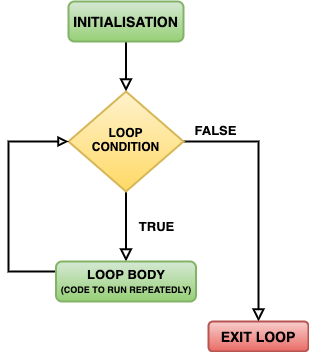### How to do While loops

This is what the template of a While loop looks like -

``````while (condition) {
# statement
}
``````

Unlike a For loop, you don’t need to specify any initialization in your While loop expression. All you need to specify is a loop condition. This loop condition usually uses an iterator variable that has already been initialized before in the code. Updating of this iterator variable usually happens near the end of the loop body.

### PHP While loop example

Let us understand this through the same example of printing the whole numbers from 0 to 5.

``````<?php

\$i = 0;

while (\$i<=5) {
echo \$i. " ";
\$i++;
}

?>``````

OUTPUT: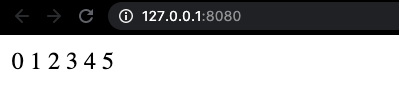## Do-While loops

Another type of loops are Do-While loops. In these loops, the loop body is defined in a do block before defining the while condition.

This is what the template of a Do-While loop looks like -

``````do {
# statement
} while (condition);``````

The most important difference between a While loop and a Do-While loop is that in a Do-While loop, the condition is always evaluated at the end of each loop. This is unlike what is done in While loops where the condition is always evaluated before running the loop body.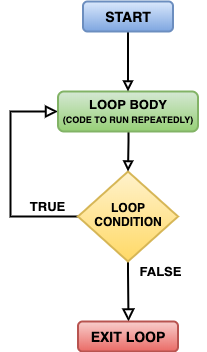This means that in a Do-While loop, the body is always going to be executed at least once even if the condition is false. This does not hold true for all other types of loops.

### PHP Do-While loop example

Let us try the same whole numbers example using a Do-While loop.

``````<?php

\$i = 0;
do {
echo \$i. " ";
\$i++;
}
while (\$i<=5);

?>``````

OUTPUT: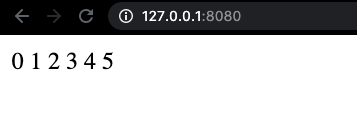## Conditional Statements in PHP

Till now we looked at different types of loops in PHP and learned how we can use them to program different iterative flows.

In this section, we will look at how we can use conditional structures in PHP.

### What are conditional statements?

Conditional statements in programming languages allow you to execute different code segments depending on the satisfaction of different conditions. It allows you to account for any uncertainty in your input or any intermediate results and handle the occurrence of multiple possibilities.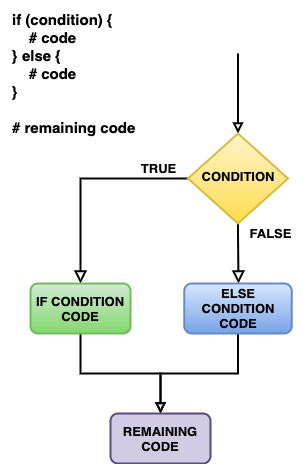### PHP If-else example

The most common approach to implementing conditional branches is using If-Else statements. Let’s understand using an example.

``````<?php

\$i = 12;
if (\$i % 2 == 0) {
echo "The number ".\$i." is even.";
} else {
echo "The number ".\$i." is odd.";
}

?>``````

OUTPUT: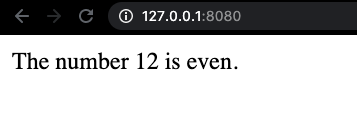You can also use elseif to define multiple branches or to handle the possibility of multiple conditions. Let’s handle the condition of the number being zero in our example above.

``````<?php

\$i = 12;
if (\$i % 2 == 0) {
echo "The number ".\$i." is even.";
} elseif (\$i == 0) {
echo "The number ".\$i." is zero.";
} else {
echo "The number ".\$i." is odd.";
}

?>``````

In this case, we only had three possibilities to check for. As the number of conditions to keep track of increases, you might want to look at another way of implementing conditional logic.

## Switch-Case Statements

Quite often, when all your if-conditions check for the different values of a variable, it can be tiring to define all the if-cases.

I think the best way to understand switch-case statements is by contrasting it with the if-else method of defining conditions. Take this example of printing the name strings of the certain integers using if-conditions -

``````<?php

\$i = 2;

if (\$i == 0) {
echo "Zero";
} elseif (\$i == 1) {
echo "One";
} elseif (\$i == 2) {
echo "Two";
} elseif (\$i == 3) {
echo "Three";
}
?>``````

This looks a little redundant as you can see. We’re having to type the equality comparison each time even though it’s the same variable (\$i) whose values we are checking.

Let’s implement the same example using switch-case statements and see how easy it can be.

``````<?php

\$i = 2;

switch (\$i) { # specifying the variable here
case 0: # specifying the value we're checking for here
echo "Zero";
break;
case 1:
echo "One";
break;
case 2:
echo "Two";
break;
case 3:
echo "Three";
break;
}
?>``````

As shown in the example, you can specify the variable in your switch expression, and the values you’re comparing against as arguments to your various case calls.

OUTPUT: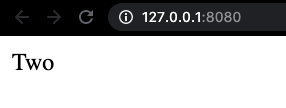In the above example, we compare our variable with integer values. We can also use switch-cases for comparing with string values.

``````<?php

\$i = "Zero";

switch (\$i) {
case "Zero":
echo 0;
break;
case "One":
echo 1;
break;
case "Two":
echo 2;
break;
}
?>``````

OUTPUT: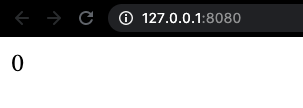As you can see, switch-case statements are essentially similar to a series of if-statements on the same expression, that can be defined a whole lot easier.

## Return statement

The last control structure that we’ll look at in this post is return

The return statement is used to transfer program control from the current block back to the calling module. It is most commonly used from inside a function. This results in the execution of the function are terminated and control is transferred to the module that called the function.

Let’s understand this through a basic example.

``````<?php

function printMessage() {
echo "Hello ..";
return;
echo " World!";
}

printMessage();

?>``````

As you can see, upon calling the printMessage() function, a return call is issued after printing the Hello string. Therefore, the remaining body of the function is not executed and we’re not able to print the other string.

OUTPUT: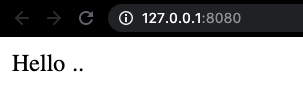Return is somewhat similar to break in the sense that it gets you out of places, but both are quite different in what they do.

## Putting it together

Now that we’re done with all the control structures, I’d highly recommend you to try your hand at programming various control flows. As I mentioned before, these control structures form the very fundamentals of programming paradigms, and one way you can get better at these is by practicing.

An all-encompassing practice example I’d suggest is writing a PHP program that uses switch-case statements that provides basic examples of each of the different types of loops and conditional statements that we read in this post. It can look something like this -

``````switch (\$i) {
case 0:
# for loop example
break;
case 1:
# for-each loop example
break;
.
.
.
}``````

## Recap

In this post, we learned about control structures in programming and their two types - Repetitive (Loop-based) structures and Conditional structures. We looked at all the different types of loops in PHP (for loops, for-each loops, while loops and do-while loops), how to break out of loops and how to continue to the next loop iteration. We also learned how to implement conditional statements using if-else and switch-case statements. I hope you learned something valuable from this post.

Stay healthy, stay home, and stay safe! Happy coding!